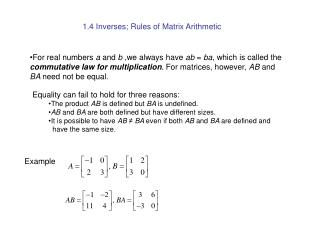Download PresentationExample

# Example

Download Presentation## Example

- - - - - - - - - - - - - - - - - - - - - - - - - - - E N D - - - - - - - - - - - - - - - - - - - - - - - - - - -
##### Presentation Transcript

1. 1.4 Inverses; Rules of Matrix Arithmetic • For real numbers a and b ,we always have ab = ba, which is called the commutative law for multiplication. For matrices, however, AB and BA need not be equal. • Equality can fail to hold for three reasons: • The product AB is defined but BA is undefined. • AB and BA are both defined but have different sizes. • It is possible to have AB ≠ BA even if both AB and BA are defined and • have the same size. Example

2. Theorem 1.4.1 (Properties of Matrix Arithmetic) Assuming that the sizes of the matrices are such that the indicated operations can be performed, the following rules of matrix arithmetic are valid: (a) A + B = B + A (commutative law for addition) (b) A + (B + C) = (A + B) + C (associative law for addition) (c) A(BC) = (AB)C (associative law for multiplication) (d) A(B + C) = AB + AC (left distributive law) (e) (B + C)A = BA + CA (right distributive law) (f) A(B – C) = AB – AC, (B – C)A = BA – CA (g) a(B + C) = aB + aC, a(B – C) = aB – aC (h) (a+b)C = aC + bC, (a-b)C = aC – bC (i) a(bC) = (ab)C, a(BC) = (aB)C = B(aC)

3. Zero Matrices A matrix, all of whose entries are zero, is called a zero matrix • A zero matrix will be denoted by 0 • If it is important to emphasize the size, we shall write for the m×n zero matrix. • In keeping with our convention of using boldface symbols for matrices with one column, we will denote a zero matrix with one column by 0 Theorem 1.4.2 (Properties of Zero Matrices) Assuming that the sizes of the matrices are such that the indicated operations can be performed ,the following rules of matrix arithmetic are valid (a) A + 0 = 0 + A = A (b) A – A = 0 (c) 0 – A = -A (d) A0 = 0; 0A = 0

4. Note: the cancellation law is not valid for matrix multiplication! Example But

5. Identity Matrices A square matrix with 1′s on the main diagonal and 0′s off the main diagonal is called an identity matrix and is denoted by I, or Infor the n×n identity matrix If A is an m×n matrix, then AIn= A and ImA = A Theorem 1.4.3 If R is the reduced row-echelon form of an n×n matrix A, then either R has a row of zeros or R is the identity matrix In

6. Definition If A is a square matrix, and if a matrix B of the same size can be found such that AB = BA = I, then A is said to be invertible and B is called an inverse of A. If no such matrix B can be found, then A is said to be singular. • Remark: • The inverse of A is denoted as • Not every (square) matrix has an inverse • An inverse matrix has exactly one inverse

7. Properties of Inverses Theorem 1.4.4 If B and C are both inverses of the matrix A, then B = C Theorem 1.4.5 The matrix is invertible if ad – bc ≠ 0, in which case the inverse is given by the formula

8. Example: Theorem 1.4.6 If A and B are invertible matrices of the same size ,then AB is invertible and

9. Definition If A is a square matrix, then we define the nonnegative integer powers of A to be n factors If A is invertible, then we define the negative integer powers to be n factors n factors

10. Theorems Theorem 1.4.7 (Laws of Exponents) If A is a square matrix and r and s are integers, then Theorem 1.4.8 (Laws of Exponents) If A is an invertible matrix, then: • is invertible and • is invertible and for n = 0, 1, 2, … • (c) For any nonzero scalar k, the matrix is invertible and

11. Polynomial Expressions Involving Matrices If A is a square matrix, say m×m , and if is any polynomial, then we define where I is the m×m identity matrix. Example

12. Properties of the Transpose Theorem 1.4.9 (Properties of the Transpose) If the sizes of the matrices are such that the stated operations can be performed, then (a) (b) (c) , where k is any scalar (d) Theorem 1.4.10 (Invertibility of a Transpose) If A is an invertible matrix, then is also invertible and